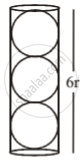# A Cylindrical Tennis Ball Container Can Contain Maximum Three Balls Stacked on One Another. the Top and Bottom Bails Also Touch the Lid and the Base of the Container Respectively. If the Volume of a - Mathematics

#### Question

MCQ

A cylindrical tennis ball container can contain maximum of three balls stacked on one another. The top and bottom bails also touch the lid and the base of the container respectively. If the volume of a tennis ball is 240 cm3, then what is the volume of the container?

• 1080 cm3

• 840 cm3

• 1440 cm3

• 720 cm3

#### Solution

1080 cm3

Explanation:

Given volume of 1 Tennis Ball = 240 cm3

VB = 4/3 pi "r"^3 = 240

"r"^3 = (240 xx 3)/(4pi)Radius of cylindrical container = r

Height of cylindrical container = 6r

Volume of cylindrical container = π r2 (6r)    {πr2h}

Vc = 6πr3

Vc = 6 × π × (240 xx 3)/(4 xx pi) = 1080 "cm"^3

Concept: Area and Volume (Entrance Exam)
Is there an error in this question or solution?